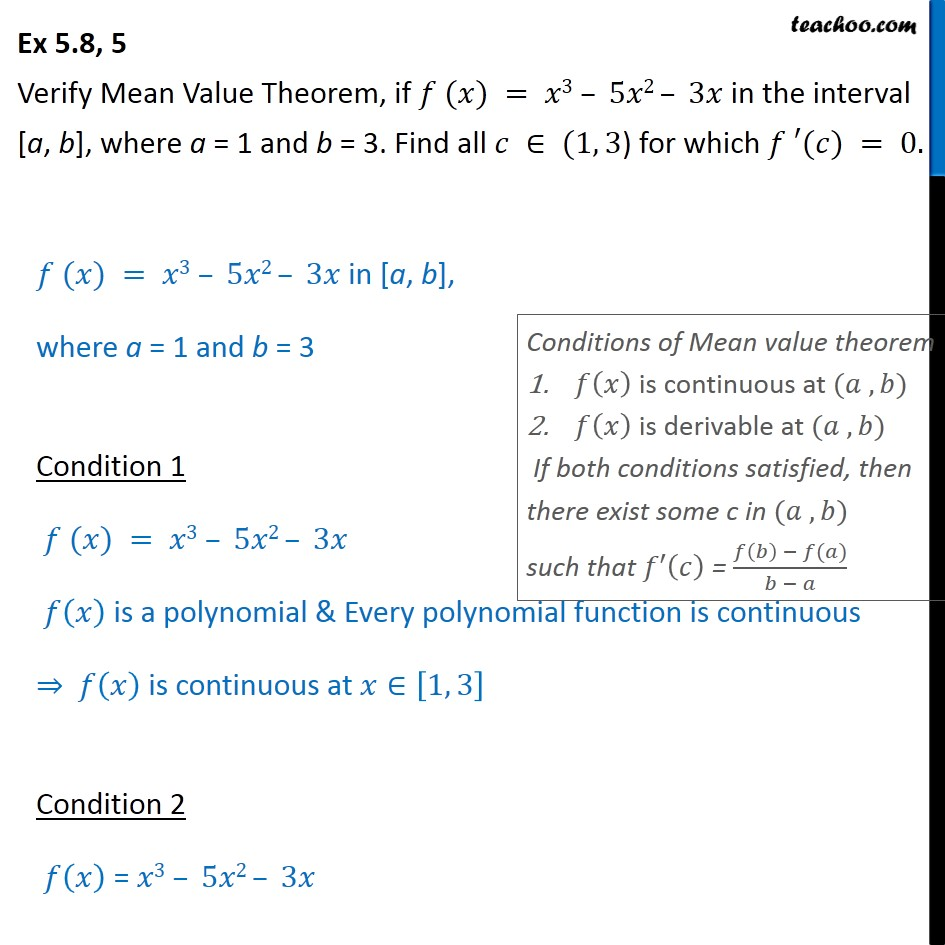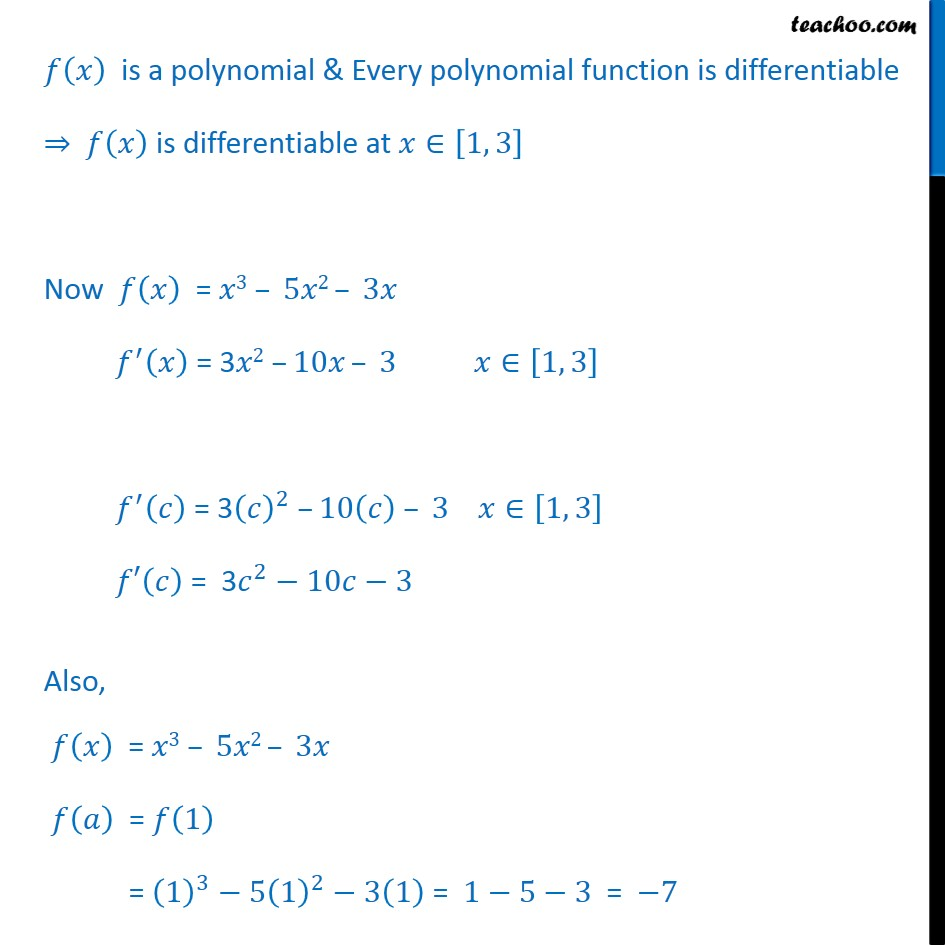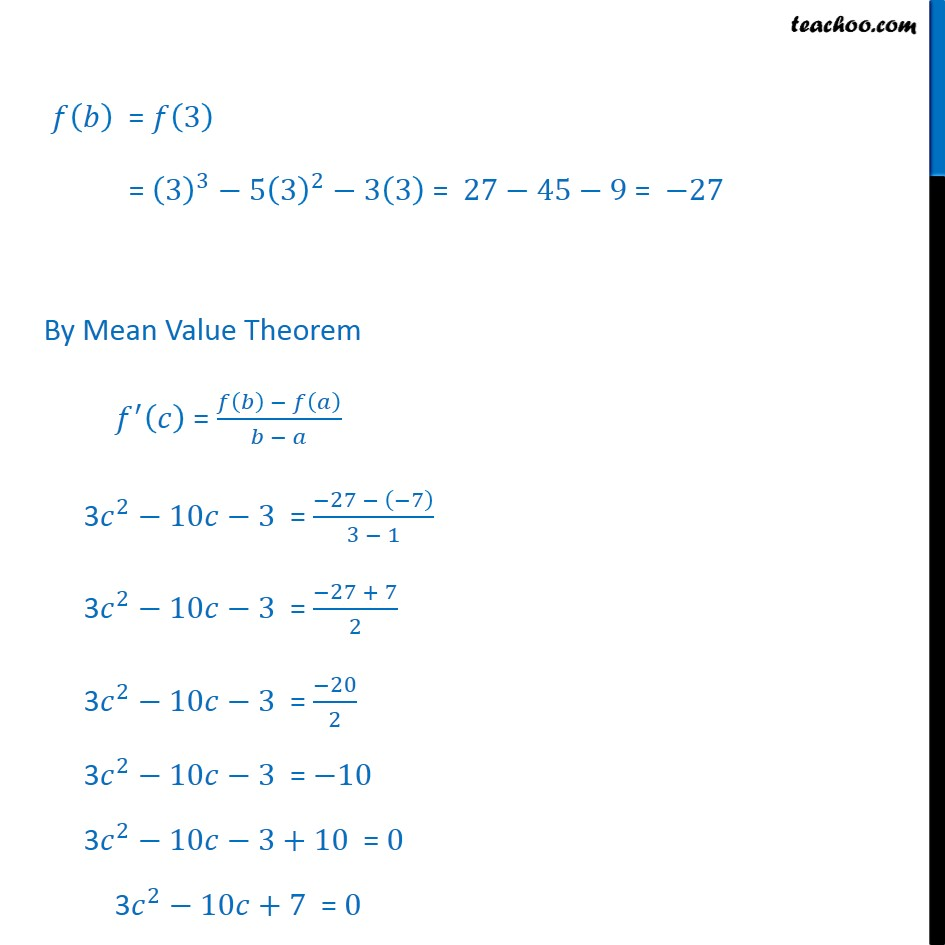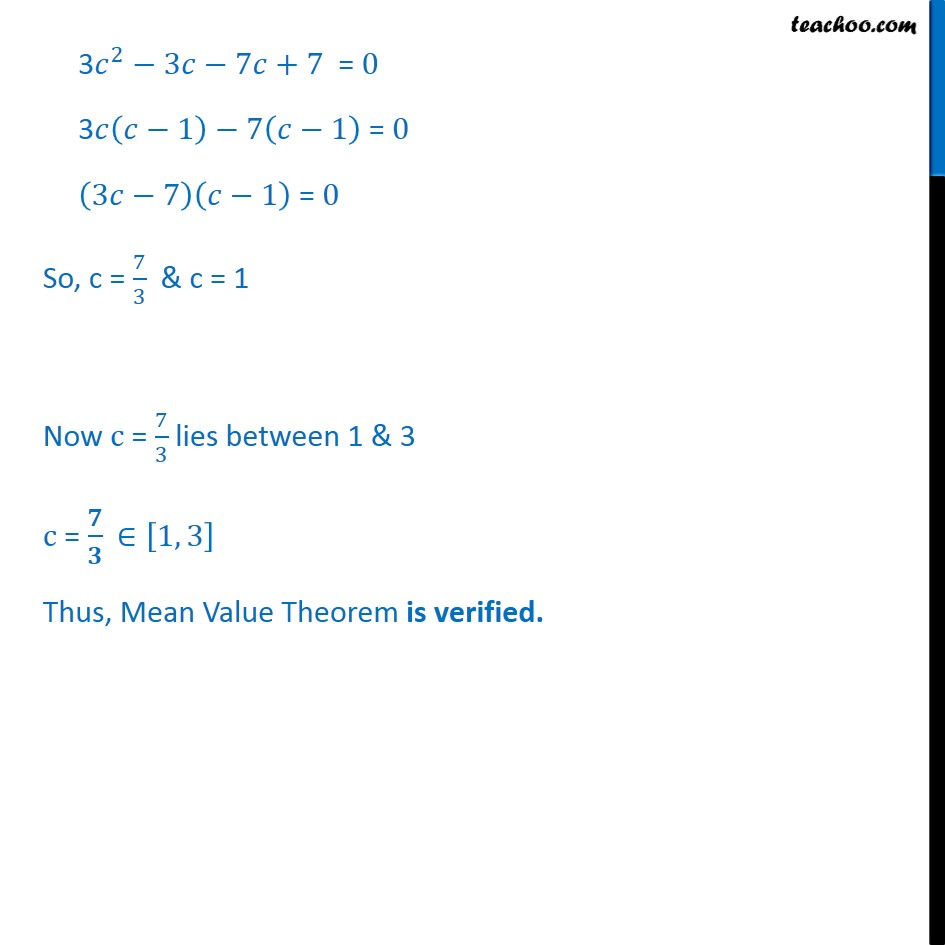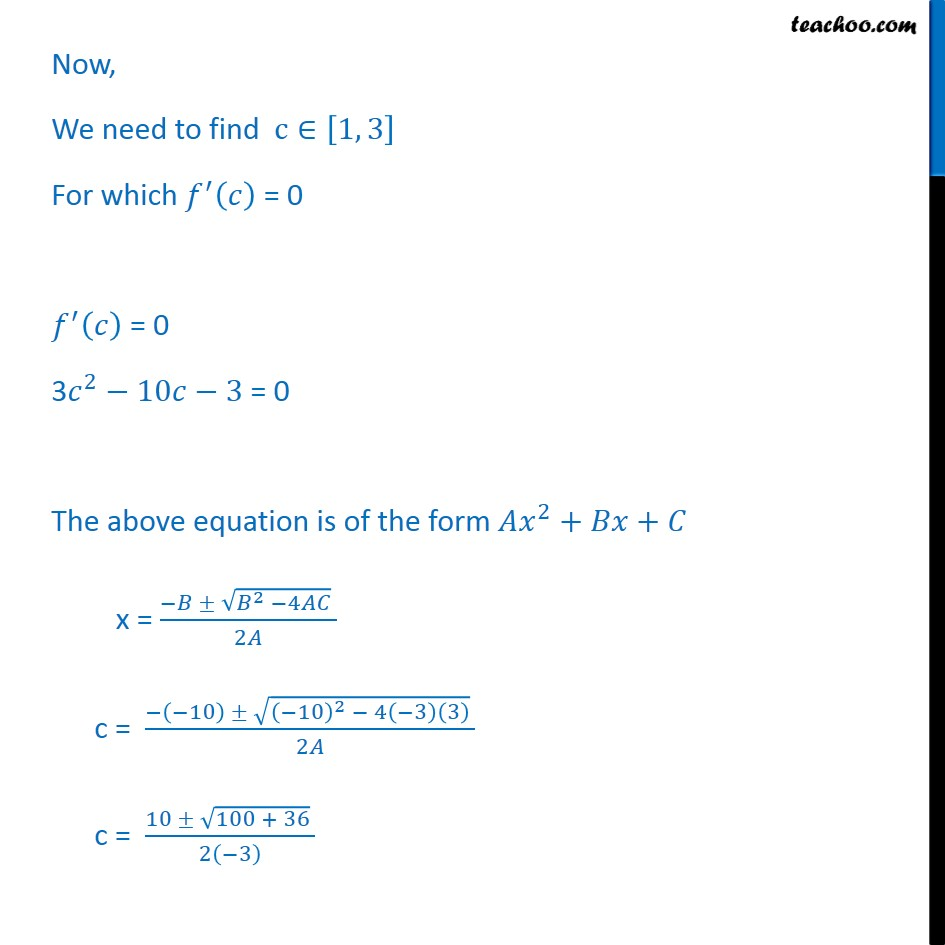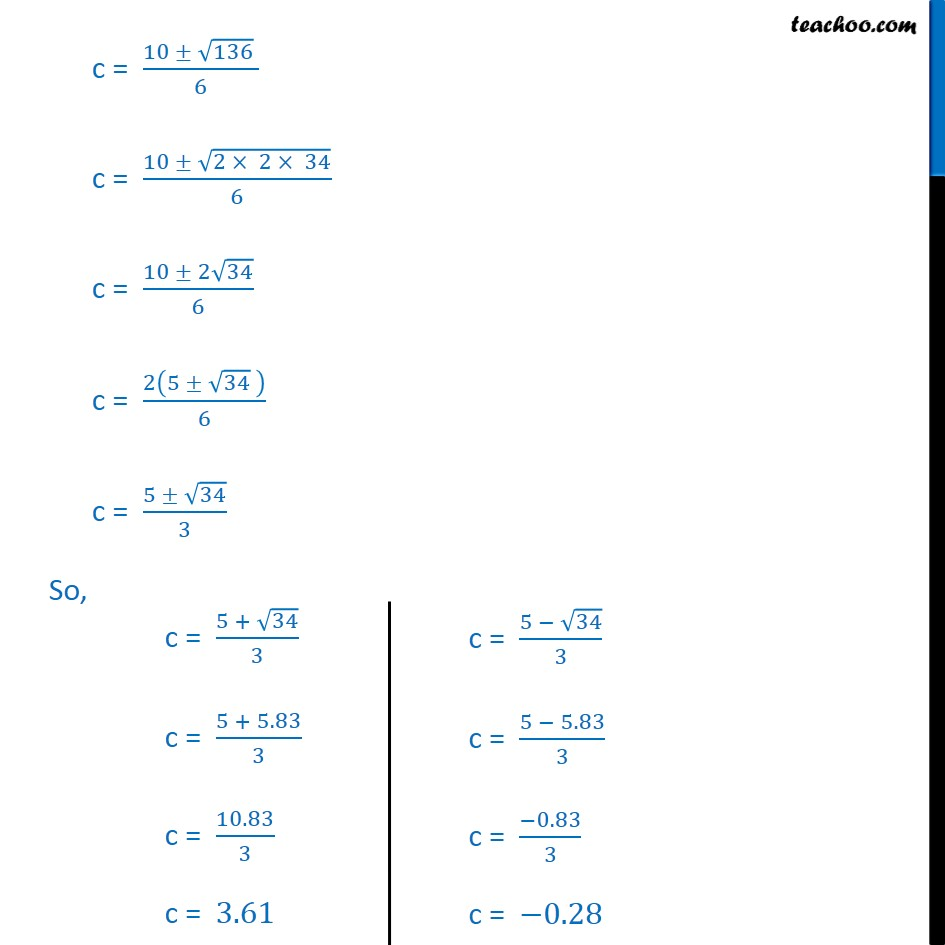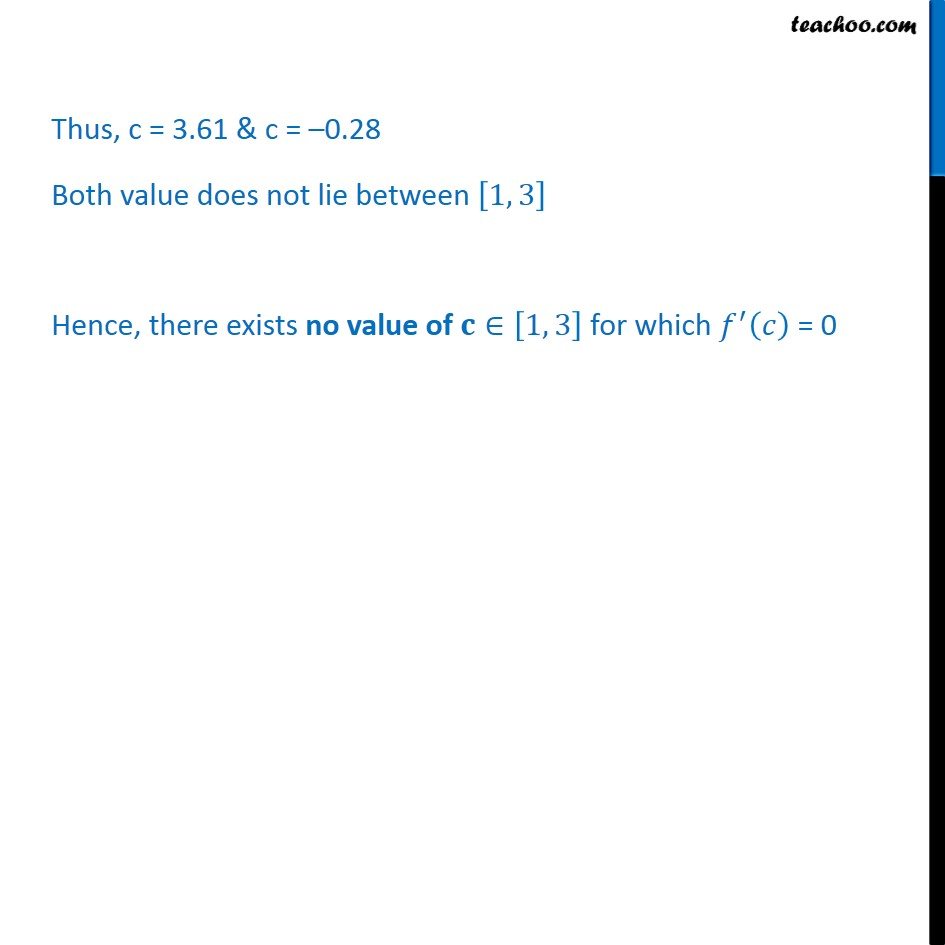1. Class 12
2. Important Question for exams Class 12
3. Chapter 5 Class 12 Continuity and Differentiability

Transcript

Ex 5.8, 5 Verify Mean Value Theorem, if 𝑓 (𝑥) = 𝑥3 – 5𝑥2 – 3𝑥 in the interval [a, b], where a = 1 and b = 3. Find all 𝑐 ∈ (1, 3) for which 𝑓 ′(𝑐) = 0. 𝑓 (𝑥) = 𝑥3 – 5𝑥2 – 3𝑥 in [a, b], where a = 1 and b = 3 Condition 1 𝑓 (𝑥) = 𝑥3 – 5𝑥2 – 3𝑥 𝑓 𝑥﷯ is a polynomial & Every polynomial function is continuous ⇒ 𝑓 𝑥﷯ is continuous at 𝑥∈ 1, 3﷯ Condition 2 𝑓 𝑥﷯ = 𝑥3 – 5𝑥2 – 3𝑥 𝑓 𝑥﷯ is a polynomial & Every polynomial function is differentiable ⇒ 𝑓 𝑥﷯ is differentiable at 𝑥∈ 1, 3﷯ Now 𝑓 𝑥﷯ = 𝑥3 – 5𝑥2 – 3𝑥 𝑓﷮′﷯ 𝑥﷯ = 3𝑥2 –10𝑥 – 3 𝑥∈ 1, 3﷯ 𝑓﷮′﷯ 𝑐﷯ = 3 𝑐﷯﷮2﷯ –10 𝑐﷯ – 3 𝑥∈ 1, 3﷯ 𝑓′ 𝑐﷯ = 3 𝑐﷮2﷯−10𝑐−3 Also, 𝑓 𝑥﷯ = 𝑥3 – 5𝑥2 – 3𝑥 𝑓 𝑎﷯ = 𝑓 1﷯ = 1﷯﷮3﷯−5 1﷯﷮2﷯−3 1﷯ = 1−5−3 = −7 𝑓 𝑏﷯ = 𝑓 3﷯ = 3﷯﷮3﷯−5 3﷯﷮2﷯−3 3﷯ = 27−45−9 = −27 By Mean Value Theorem 𝑓﷮′﷯ 𝑐﷯ = 𝑓 𝑏﷯ − 𝑓 𝑎﷯﷮𝑏 − 𝑎﷯ 3 𝑐﷮2﷯−10𝑐−3 = −27 − −7﷯﷮3 − 1﷯ 3 𝑐﷮2﷯−10𝑐−3 = −27 + 7﷮2﷯ 3 𝑐﷮2﷯−10𝑐−3 = −20﷮2﷯ 3 𝑐﷮2﷯−10𝑐−3 = −10 3 𝑐﷮2﷯−10𝑐−3+10 = 0 3 𝑐﷮2﷯−10𝑐+7 = 0 3 𝑐﷮2﷯−3𝑐−7𝑐+7 = 0 3𝑐 𝑐−1﷯−7 𝑐−1﷯ = 0 3𝑐−7﷯ 𝑐−1﷯ = 0 So, c = 7﷮3﷯ & c = 1 Now c = 7﷮3﷯ lies between 1 & 3 c = 𝟕﷮𝟑﷯ ∈ 1, 3﷯ Thus, Mean Value Theorem is verified. Now, We need to find c∈ 1, 3﷯ For which 𝑓﷮′﷯ 𝑐﷯ = 0 𝑓﷮′﷯ 𝑐﷯ = 0 3 𝑐﷮2﷯−10𝑐−3 = 0 The above equation is of the form 𝐴 𝑥﷮2﷯+𝐵𝑥+𝐶 x = −𝐵 ± ﷮ 𝐵﷮2﷯ −4𝐴𝐶﷯ ﷮2𝐴﷯ c = − −10﷯ ± ﷮ −10﷯﷮2﷯ − 4 −3﷯ 3﷯﷯ ﷮2𝐴﷯ c = 10 ± ﷮100 + 36﷯ ﷮2 −3﷯﷯ c = 10 ± ﷮136﷯ ﷮6﷯ c = 10 ± ﷮2 × 2 × 34﷯﷮6﷯ c = 10 ± 2 ﷮34﷯﷮6﷯ c = 2 5 ± ﷮34﷯ ﷯﷮6﷯ c = 5 ± ﷮34﷯﷮3﷯ So, Thus, c = 3.61 & c = –0.28 Both value does not lie between 1, 3﷯ Hence, there exists no value of 𝐜∈ 1, 3﷯ for which 𝑓﷮′﷯ 𝑐﷯ = 0

Chapter 5 Class 12 Continuity and Differentiability

Class 12
Important Question for exams Class 12